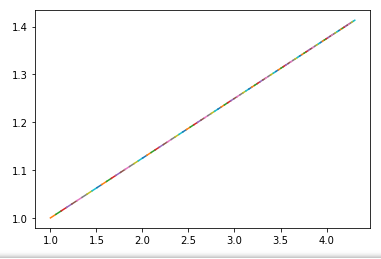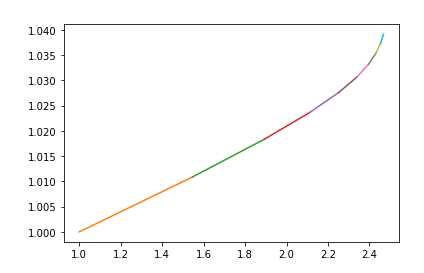# Full Batch Gradient Descent Issue

I’m trying to implement full batch gradient descent with the new fastai library for a blog post I’m writing. I think I’m close to something that will work, but I think I’m slightly incorrect currently. So I tried taking `self._backward()`, `self._step()`, and `self.opt.zero_grad()` and put them into `learn._do_epoch` rather than `learn._do_one_batch` but I am never getting actual updates. Here is what my two functions look like now if anybody sees any issues with my code:

``````def _do_epoch_BGD(self):
self._do_epoch_train()
if not self.training or not len(self.yb): return
self('before_backward')
self._backward()
self('after_backward')
self._step()
self('after_step')
self._do_epoch_validate()
``````
``````def _do_one_batch_BGD(self):
self.pred = self.model(*self.xb)
self('after_pred')
if len(self.yb): self.loss = self.loss_func(self.pred, *self.yb)
self('after_loss')
``````
``````model = MySimpleModel()
loss_func = mse #F.mse_loss
𝜂 = 0.0001
partial_learner = partial(Learner,dls, model, loss_func, cbs=[OptimizerInsight])
learn = partial_learner(SGD, lr=𝜂)
learn._do_epoch = partial(_do_epoch_BGD,learn)
learn._do_one_batch = partial(_do_one_batch_BGD,learn)
``````

But when I try actually running fit:

``````learn.fit(100)
``````

I get this result:It seems like it use the same gradients no matter what.

I was able to solve this issue. Basically my issues were misunderstanding how everything was implemented. I needed to do my backwards computation in the one_batch still, but only do my step and zero_grad steps up in _do_epoch. Here is the code that I believe is working now:

``````def _do_epoch_BGD(self):
self._do_epoch_train()
self._step()
self('after_step')
self._do_epoch_validate()
``````
``````def _do_one_batch_BGD(self):
self.pred = self.model(*self.xb)
self('after_pred')
if len(self.yb): self.loss = self.loss_func(self.pred, *self.yb)
self('after_loss')
if not self.training or not len(self.yb): return
self('before_backward')
self._backward()
self('after_backward')
``````

Here is what my result looks like now:2 Likes